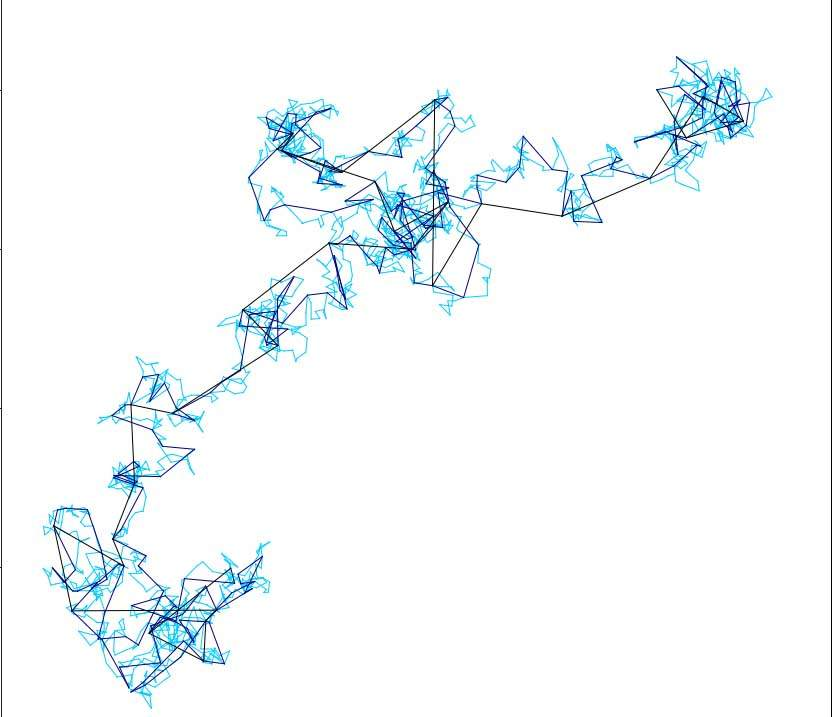# Taming the Curse of High Dimensionality

## You are here

### TagsWhen mathematicians talk about spatial dimensions, they usually mean the number of coordinates needed to describe a point or sample in that space. The universe as we know it has three dimensions. But higher-dimensional spaces are needed to describe, for example, all the possible states of a system with 100 particles, in which each particle is described by location and velocity. That system will have 100 x 3 x 2, or 600 dimensions. Or one could try to visualize the space of all possible pictures on a screen 1,000 x 1,000 pixels. Taking into account the three colors used to describe each pixel, that space would have three million dimensions. The dimensions of a space containing all possible human DNA sequences numbers in the hundreds of millions.

In today’s era of “big data,” scientists often struggle with datasets that have large numbers of dimensions. In three-dimensional space, we can visualize how a set of points having the same distance from a given point creates a sphere. But how, for example, can we visualize the set containing all pictures of dogs? Or what about an array of blood tests, each containing some 100 measurements, in which we want to identify the combination of factors that represents a heightened risk for diabetes? Can we say something about what the geometry of such high-dimensional information would look like?

In these examples, a central problem is that the number of possibilities quickly becomes astronomical. Even if we limited the pixels to either black or white and the screen resolution was low, the number of possible images is immense. That is, if we must scan all the points in this space (in this case, all possible combinations of pixels), we end up with a number that grows exponentially with the dimensions. This phenomenon is often called the curse of dimensionality.

## More variables, less randomness

Dr. Ronen Eldan of the Weizmann Institute of Science’s Mathematics Department is one of those who are working to overcome the curse of dimensionality. A relatively new mathematical theory shows that in many cases, high-dimensional systems can be reduced to something more manageable. If we look at such a system in the right way, certain simple structures can be found within the seeming disarray. Eldan recently received the Erdös Prize awarded by the Israel Mathematical Union for his work in high-dimensional mathematics.

The number of possibilities quickly becomes astronomical

One of the basic ideas underlying this field of math is that of averages, or the “law of large numbers.” According to this law, as the number of variables in a system grows, the average of all those variables will be less random. This can be applied to the stock market, for example: If we take the average of all the daily percentage changes over enough time, we will eventually arrive at a number that is nearly constant (or almost invariable). This is the foundation of the “concentration of measure” pioneered by, among others, Prof Vitali Milman of Tel Aviv University.

This basic idea has inspired a theory leading to numerous techniques that facilitate data analysis. For example, in the case of photos, it turns out that rather than measuring each pixel separately, it is worthwhile to randomly group together pixels and average them before carrying out the analysis. Similar methods can also be applied to many kinds of large datasets.

## The diffusion-dimension connection

“High dimensional systems appear in statistics, computer science and physics, as well as in various types of machine learning,” says Eldan. One of the ways he is addressing the problem is to look for motifs – things that repeat themselves in different systems. These motifs, he says, may represent deep properties that are common to many high-dimension systems; it can sometimes be surprising how one often finds the same phenomenon in two seemingly unrelated areas. Eldan, for example, discovered unexpected connections between the behavior of certain types of high-dimensional systems and Brownian motion, which describes the diffusion of tiny particles in a fluid. A mathematical model of Brownian motion named the Wiener process is used to describe phenomena in fields ranging from physics to biology and economics.Eldan is developing a new analytical method for the analysis of high-dimensional systems based on the models of Brownian motion and diffusion, which, as well as providing new tools may lead to further insights into the common properties of these systems. In another series of studies, Eldan is focusing on understanding how high-dimensional theory can aid in the development of algorithms for optimization and machine learning. Ultimately, he says, dimensionality is both a curse and a blessing. The diversity that comes with it may complicate the process of analysis; but it often comes together with hidden structure if we are able to look at it from the correct point of view. It is that point of view, highly multidimensional and hard to visualize, that Eldan is helping bring into focus.

Dr. Ronen Eldan is the incumbent of the The Elaine Blond Career Development Chair in Perpetuity.

### Tags: